# Semiconductors

## Semiconductors

The following text is used only for teaching, research, scholarship, educational use and informative purpose following the fair use principles.

We thank the authors of the texts and the source web site that give us the opportunity to share their knowledge

# Semiconductors

A Semiconductor is a material whose resistivity is between that of a good conductor and a good insulator.
Examples of materials which are semiconductors are Silicon and Germanium.

The resistance of a semiconductor decreases as its temperature increases*.

Intrinsic Conduction is the movement of charges through a pure semiconductor.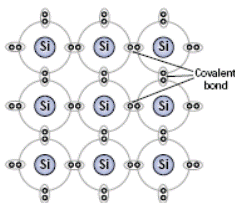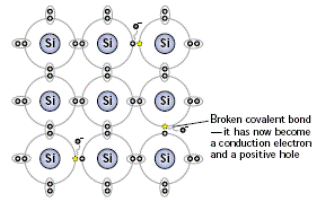A Thermistor is an electrical component whose resistance decreases rapidly with increasing temperature.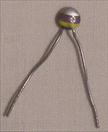Symbol for a thermistor:Component Symbol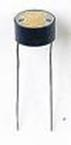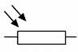A Light Dependant Resistor (LDR) is an electrical component whose resistance decreases rapidly when light shines on it.

Doping
Doping is the addition of a small amount of atoms of another element to a pure semiconductor to increase its conductivity*.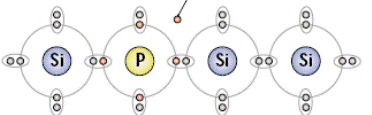Extrinsic Conduction is the movement of charges through a doped semiconductor.

An n-type semiconductor is a semiconductor in which electrons are the majority charge carriers.

• In this case there is one ‘extra’ electron due to the addition of the Phosphoros atom.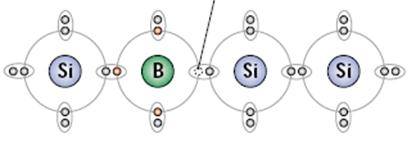A p-type semiconductor is a semiconductor in which holes are the majority charge carriers.

• In this case there is one ‘missing’ electron due to the addition of the Boron atom. This is equivalent to a ‘Positive Hole’ which moves in the opposite direction to an electron.

The operation of semiconductor devices depends on the effects that occur when p-type and n-type semiconductor material are in close contact. This is achieved by taking a single crystal of silicon and doping separate but adjacent layers of it with suitable impurities. The junction between the p-type and the n-type layers is referred to as the p–n junction and this is the key to some very important aspects of semiconductor theory.

Devices such as diodes, transistors, silicon-controlled rectifiers, etc., all contain one or more p–n junctions.

The p-n junction*
When a piece of p-type semiconductor is joined to a piece of n-type semiconductor, the junction between the two is known as a p-n junction.

The Depletion Region
The depletion region is so named because it is formed from a conducting region of the semiconductor which has been depleted of all free charge carriers, leaving none to carry a current.
Understanding the depletion region is key to explaining modern semiconductor electronics in action.

• Due to thermal agitation, some free electrons in the n-type material diffuse over to the p-type material, where they combine with nearby positive holes, with the result that the region is depleted of two of its charge carriers.
• Similarly on the p-type side some positive holes diffuse over to the n-type material, where they too combine with nearby electrons, with the result that the region gets depleted of two more of its charge carriers.
• The end result is that a depletion region is formed at the junction of the p-type and n-type materials, where there are no free charge carriers. This region therefore acts as an insulator.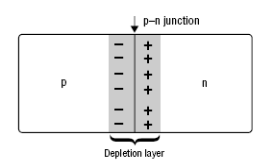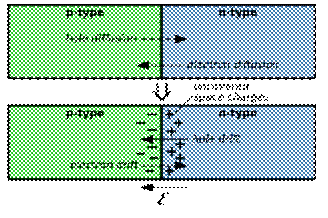• Work now needs to be done to bring charge from one side of this depletion region to the other; therefore a potential difference (voltage) exists across the region.
• This voltage is typically about 0.1 volts for a germanium diode, and 0.6 volts for a silicon diode.
• This voltage is known as the junction voltage.

Current flow across a p-n junction
Forward-biased p-n junction

•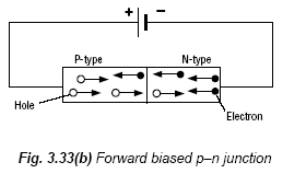Here the positive terminal of the junction is connected to the p-type and the negative terminal is connected to the n-type.
• Electrons in the N-type area above are repelled from the negative terminal and drive in to the depletion region.
• Similarly positive holes in the P-type are repelled from the positive terminal and drive into the depletion region from the other side.
• The end result is that the width of the depletion layer is reduced.
• In fact if the voltage across the terminals is sufficiently great (greater than what is known as the junction voltage) the depletion layer is completely broken down and the region now becomes a conductor.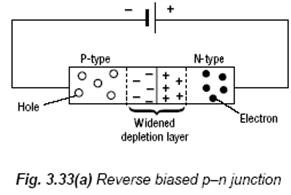Reversed-biased p-n junction

• Here the positive terminal of the junction is connected to the n-type and the negative terminal is connected to the p-type.
• Electrons in the N-type area above are now attracted to the positive terminal and because as a result the depletion region in this section grows bigger.
• Similarly positive holes in the P-type are attracted to the negative terminal.
• The end result is that the width of the depletion layer is increased.
• The end result is that no charges actually move through the depletion region and it remains an insulator.

Applications of the p-n junction
The p-n junction is the principle behind the semiconductor diode, which lies at the heart of most electronic systems, including LEDs, computers and integrated circuits.
It is also responsible for rectification of a.c. (converting a.c. to d.c.).*

A (semiconductor) diode will only allow current to flow in one direction.

 Image Symbol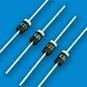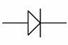Graph of Current against Voltage for a Semiconductor diode.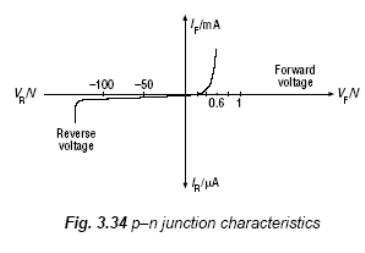• The left hand side of the graph represents the p-n junction in reverse bias and little or no current flows.
• At –100 volts, the junction gets destroyed.
• In forward bias (the right hand side) very little current flows until the voltage reaches 0.6 Volts (junction voltage).
• At this stage the depletion layer gets completely broken down and the region now becomes a conductor, and therefore the current rises suddenly.

A diode is a device that allows current to flow in one direction only.

The rectifier is a device that uses diodes to convert a.c. to d.c
Examples of appliances that contain a rectifier: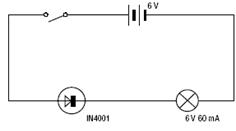Radio, television, computer, battery charger, mobile phone charger.

Demonstrating the action of a diode
Use a light bulb in series with the semiconductor and the battery.
Observation: Bulb lights when switch is closed (circuit is forward biased), but not when the diode is put in the other way around (reverse biased).

 Image Symbol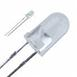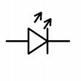The Light-Emitting Diode (LED)

An LED consists of a junction diode made from semiconducting material.
Light is emitted from the junction when it is forward biased and the brightness is approximately proportional to the forward current.

Mandatory Experiments

• To investigate how Resistance changes with Temperature for a Thermistor
• To investigate the variation of Current with Potential Difference for a Semiconductor Diode

Leaving Cert Physics Syllabus

 Content Depth of Treatment Activities STS Conduction in materials Conduction in semiconductors. References to charge carriers. Interpretation of I–V graph. Conduction in semiconductors: the distinction between intrinsic and extrinsic conduction; p-type and n-type semiconductors. Electronic devices. LED, computers, integrated circuits. The p-n junction: basic principles underlying current flow across a p-n junction. Demonstration of current flow across a p-n junction in forward and reverse bias, e.g. using a bulb. Rectification of a.c. LDR – light-dependent resistor. Thermistor. Demonstration of LDR and thermistor.

TO INVESTIGATE THE VARIATION OF CURRENT (I) WITH P.D. (V) FOR A SEMICONDUCTOR DIODE IN FORWARD BIAS

APPARATUS:
Low voltage power supply, rheostat, voltmeter, ammeter, 330 Ω resistor, semiconductor diode

DIAGRAM: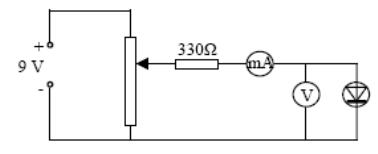PROCEDURE:
1. Set up the circuit with the semiconductor diode in forward bias as shown.

2. Adjust the potential divider to obtain different values for the voltage V and hence for the current I.

3. Obtain at least ten values for V and for I using the voltmeter and the ammeter.

4. Plot a graph of I against V and join the points in a smooth, continuous curve.

RESULTS:

 V (volts) I (milli-amps)

CONCLUSION:
We can see from the graph that almost no current flows until the applied voltage exceeds 0.6 V for a silicon diode but then the current rises rapidly.

NOTE:
A protective resistor, e.g. 330 Ω, should always be used in series with a diode in forward bias. Therefore even if the diode offers no resistance there will still be some other resistance in the circuit preventing the current getting too high.

A germanium diode, e.g. OA91, gives very little current between 0 and 0.2 V but the current then increases rapidly above this voltage.
A light emitting diode gives very little current up to 1.6 V but then the current rises rapidly accompanied by the emission of light.

TO INVESTIGATE THE VARIATION OF CURRENT (I) WITH P.D. (V) FOR A SEMICONDUCTOR DIODE IN REVERSE BIAS

APPARATUS:
Low voltage power supply, rheostat, voltmeter, ammeter, semiconductor diode.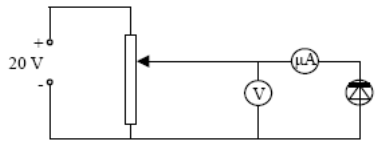DIAGRAM:

PROCEDURE:

• Set up the circuit as above and set the voltage supply at 20 V.
• The micro-ammeter is used in this part of the experiment, as current values will be very low when a diode is in reverse bias.

• Adjust the potential divider to obtain different values for the voltage V and hence for the current I.
• Obtain at least six values for V (0-20 V) and for I using the voltmeter and the micro-ammeter. Higher voltage values are required for conduction in reverse bias.

• Plot a graph of I against V and join the points in a smooth, continuous curve.

RESULTS:

 V (volts) I (micro-amps)

CONCLUSION:
We found no current in reverse bias. In theory there should be a very small amount of current but it was too small to be detected with our equipment.

PRECAUTIONS / SOURCES OF ERROR:

• Use a microammeter when the diode is reverse biased as the current flowing is extremely small.
• Change the positions of the microammeter and voltmeter (reverse biased) so that the current flowing through the diode is the only one registered in the microammeter (not current through the voltmeter)

NOTES:
The position of the voltmeter has changed since a reverse biased diode has a very large resistance that is greater than the resistance of most voltmeters. It is essential that the micro-ammeter reads only the current flowing through the reverse biased diode as the sum of the currents flowing through the voltmeter and reverse biased diode may be much larger.
Since the resistance of the micro-ammeter is negligible compared with the resistance of the reverse biased diode the potential difference across the micro-ammeter and diode is almost the same as the potential difference across the diode alone.

When using a silicon diode it is very difficult to detect the current in reverse bias as the current is so small and changes very little with temperature variations.

For a germanium diode some reverse current can be detected (a few μA) at 4 V reverse bias. This conduction increases rapidly when the diode is heated (by hand).

TO INVESTIGATE THE VARIATION OF THE RESISTANCE OF A THERMISTOR WITH TEMPERATURE

APPARATUS:
Thermistor, glycerol, beaker, heat source, thermometer, ohmmeter, boiling tube

DIAGRAM: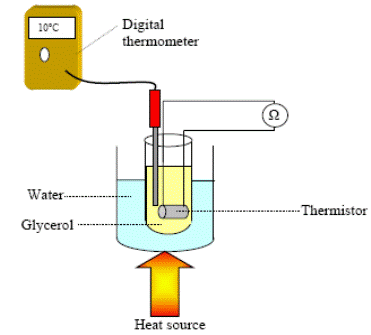PROCEDURE:
1. Set up the apparatus as shown in the diagram.

2. Use the thermometer to note the temperature of the glycerol, which we assume to be the same as the temperature of the thermistor.

3. Record the resistance of the thermistor using the ohmmeter.

4. Heat the beaker and for each 10 °C rise in temperature record the resistance and temperature using the ohmmeter and the thermometer.

5. Plot a graph of resistance against temperature.

RESULTS:

 R (W) θ (0C)

CONCLUSION:

• From the graph we can see that as temperature increases the resistance decreases.
• We believe our data to be reliable because it resulted in a smooth curve, which the theory predicted.

PRECAUTIONS / SOURCES OF ERROR:

• Check for the resistance of the connecting leads and contacts on the ohmmeter. Subtract from later readings.
• Heat very slowly to try to maintain thermal equilibrium between the water and glycerol and thermistor. When the bunsen is removed wait until the temperature is steady before taking the resistance readings.
• Use glycerol in the test tube as it is a better heat conductor than water.

Extra Credit
*The resistance of a semiconductor decreases as its temperature increases.
This is because as the temperature of the material increases it heats up, releasing many electrons from their atoms.
These electrons are now available for conduction and so resistance decreases.
This ‘liberation’ of electrons can also be caused by light shining on the material.

*Doping is the addition of a small amount of atoms of another element to a pure semiconductor to increase its conductivity.
You don’t have to know how this works but an explanation is given on page 287.
Note that the overall charge of the material is still zero.

*It is also responsible for rectification of a.c.
A forward-based diode conducts current.
A reversed-biased diode does not conduct current.
The semiconductor diode can therefore be used as a rectifier.
A rectifier is an electrical component which converts alternating current (a.c.) to direct current (d.c.).
Take any appliance that you have at home which seems to operate both on mains electricity and by battery.
The appliance will only work by direct current, so if there are no batteries and the appliance is plugged into the ‘mains’, which is a.c. there must be a rectifier in your appliance which converts the a.c. to d.c.
It also needs to have a transformer which drops the voltage from 240 volts down to whatever voltage the combination of batteries would supply.
Next time you are about to throw one of these out, take off the back and see if you identify these two components (the transformer is usually positioned just at the point where the voltage goes in).
We will look at these in more detail at the end of the chapter on Electromagnetic Induction.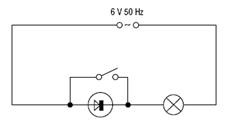Test yourself
Set up the following circuit.
1. Switch on the power and note that the lamp lights.

2. Close the switch and note that the lamp glows more brightly.

Why? (hint: note the supply voltage).

Electron Band Structure in Germanium, My Ass
Abstract
The exponential dependence of resistivity on temperature in germanium is found to be a great big lie.
My careful theoretical modelling and painstaking experimentation reveal that:
My equipment is crap, as are all the available texts on the subject and
That this whole exercise was a complete waste of my time.

Introduction
Electrons in germanium are confined to well-defined energy bands that are separated by "forbidden regions" of zero charge-carrier density.
You can read about it yourself if you want to, although I don't recommend it.
You'll have to wade through an obtuse, convoluted discussion about considering an arbitrary number of non-coupled harmonic-oscillator potentials and taking limits and so on.
The upshot is that if you heat up a sample of germanium, electrons will jump from a non-conductive energy band to a conductive one, thereby creating a measurable change in resistivity.
This relation between temperature and resistivity can be shown to be exponential in certain temperature regimes by waving your hands and chanting "to first order".

Experiment procedure
I sifted through the box of germanium crystals and chose the one that appeared to be the least cracked.
Then I soldered wires onto the crystal in the spots shown in figure 2b of Lab Handout 32.
Do you have any idea how hard it is to solder wires to germanium?
I'll tell you: real goddamn hard.
The solder simply won't stick, and you can forget about getting any of the grad students in the solid state labs to help you out.

Once the wires were in place, I attached them as appropriate to the second-rate equipment I scavenged from the back of the lab, none of which worked properly.
I soon wised up and swiped replacements from the well-stocked research labs.
This is how they treat undergrads around here: they give you broken tools and then don't understand why you don't get any results.

In order to control the temperature of the germanium, I attached the crystal to a copper rod, the upper end of which was attached to a heating coil and the lower end of which was dipped in a thermos of liquid nitrogen.
Midway through the project, the thermos began leaking.
That's right: I pay a cool ten grand a quarter to come here, and yet they can't spare the five bucks to ensure that I have a working thermos.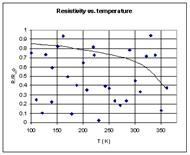Fig. 1: Check this shit out.

Results
Check this shit out (Fig. 1). That's bonafide, 100%-real data, my friends.
I took it myself over the course of two weeks.
And this was not a leisurely two weeks, either; I busted my ass day and night in order to provide you with nothing but the best data possible.
Now, let's look a bit more closely at this data, remembering that it is absolutely first-rate.
Do you see the exponential dependence?
I sure don't.
I see a bunch of crap.
Christ, this was such a waste of my time.
Banking on my hopes that whoever grades this will just look at the pictures, I drew an exponential through my noise.
I believe the apparent legitimacy is enhanced by the fact that I used a complicated computer program to make the fit.
I understand this is the same process by which the top quark was discovered.

Conclusion
Going into physics was the biggest mistake of my life.
I should've declared CS (Computer Science).
I still wouldn't have any women, but at least I'd be rolling in cash.

Taken from http://www.cs.wisc.edu/~kovar/hall.html

Exam Questions

• [2003 OL][2006 OL][2009 OL]

What is a semiconductor?

• [2003 OL][2006 OL]

Name a material used in the manufacture of semiconductors.

• 

Why is silicon a semiconductor?

• [2002 OL]

Give one difference between conduction in metals and conduction in semiconductors.

• 

As the temperature of a room increases, explain why the resistance of a thermistor decreases.

• [2002 OL]

Give two uses of semiconductors.

• [2005 OL]

Give a use for a thermistor.

• [2003 OL][2009 OL][2010 OL]

What is doping?

• 

Distinguish between intrinsic conduction and extrinsic conduction in a semiconductor.

• [2002 OL]

Semiconductors can be made p-type or n-type. How is a semiconductor made p-type?

• 

Explain how the presence of phosphorus and boron makes silicon a better conductor.

• [2003 OL]

Give one difference between a p-type semiconductor and an n-type semiconductor.

• [2003 OL]

What are the charge carriers when an electric current passes through a semiconductor?

•  [2003 OL][2006 OL]

What is a p-n junction?

• [2002 OL]

Draw a diagram showing a p-n junction connected in forward bias to a d.c. supply.

• 

What happens at the boundary of the two adjacent layers?.

• [2009 OL]

A p-n junction is formed by taking a single crystal of silicon and doping separate but adjacent layers of it.
A depletion layer is formed at the junction.
Explain how a depletion layer is formed at the junction.

• 
• Describe what happens at the boundary when the semiconductor diode is forward biased.
• Describe what happens at the boundary when the semiconductor diode is reverse biased.

• 

Why does the p-n junction become a good conductor as the potential difference exceeds 0.6 Volts?

•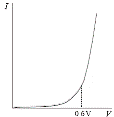Sketch a graph to show the variation of current with potential difference for a semiconductor diode in forward bias.

• 

The graph shows the variation of current I with potential difference V for a p-n junction in forward bias.
Explain, using the graph, how the current varies with the potential difference.

•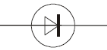[2005 OL]

Name the electrical component represented in the diagram.

• [2003 OL]

What is a diode?

• 

Give a use of a semiconductor diode.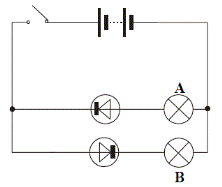• [2003 OL]

Give an example of a device that contains a rectifier.

• [2006 OL] [2009 OL]
• The circuit diagram shows 2 semiconductor diodes and 2 bulbs, labelled A and B,connected to a 6 V d.c. supply. What is observed when the switch is closed?
• Explain why.
•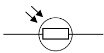[2007 OL]

Name the electrical component represented in the diagram.

Mandatory Experiments

• 

In an experiment to investigate the variation of the resistance R of a thermistor with its temperature θ, a student measured its resistance at different temperatures.

 θ /°C 20 30 40 50 60 70 80 R/Ω 2000 1300 800 400 200 90 40

The table shows the measurements recorded.

• Draw a labelled diagram of the apparatus used.
• How was the resistance measured?
• Describe how the temperature was varied.
• Using the recorded data, plot a graph to show the variation of the resistance of a thermistor with its temperature.
• Use your graph to estimate the average variation of resistance per Kelvin in the range 45 °C – 55 °C.
• In this investigation, why is the thermistor usually immersed in oil rather than in water?

•

In an experiment to investigate the variation of the resistance R of a thermistor with its temperature θ, a student measured the resistance of the thermistor at different temperatures.
The table shows the measurements recorded by the student.

 θ/ 0C 20 30 40 50 60 70 80 R/Ω 2000 1300 800 400 200 90 40
• Draw a labelled diagram of the apparatus used in this experiment.
• How did the student measure the resistance of the thermistor?
• Plot a graph on graph paper to show the relationship between the resistance R of the thermistor and its temperature θ (put θ on the X-axis).
• Use your graph to estimate the temperature of the thermistor when its resistance is 1000 Ω.
• What does your graph tell you about the relationship between the resistance of a thermistor and its temperature?

• [2002 OL]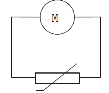The circuit diagram shows a thermistor connected to a meter M.
A student used the circuit to measure the resistance R of the thermistor at different temperatures θ.

• Name the meter M used to measure the resistance of the thermistor.
• Explain, with the aid of a labelled diagram, how the student varied the temperature of the thermistor.
• How did the student measure the temperature of the thermistor?
• The table shows the measurements recorded by the student.
 q/ 0C 20 30 40 50 60 70 80 90 R/ Ω 1300 900 640 460 340 260 200 150

Draw a graph on graph paper of resistance R against temperature θ. Put temperature on the horizontal axis.

• Using your graph, estimate the temperature of the thermistor when the meter M read 740 Ω.

•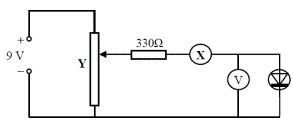[2008 OL]

The diagram shows a circuit used to investigate the variation of current with potential difference for a semiconductor diode in forward bias.

• Name the apparatus X. What does it measure?
• Name the apparatus Y. What does it do?
• What is the function of the 330 Ω resistor in this circuit?

 potential difference/V 0 0.2 0.4 0.6 0.8 1 current/mA 0 3 6 14 50 100
• The table shows the values of the potential difference used and its corresponding current recorded during the experiment.

Using the data in the table, draw a graph on graph paper of the current against the potential difference.
Put potential difference on the horizontal axis (X-axis).

• What does the graph tell you about the variation of current with potential difference for a semiconductor diode?

• 

The following is part of a student’s report of an experiment to investigate of the variation of current I with potential difference V for a semiconductor diode.
I put the diode in forward bias as shown in the circuit diagram. I increased the potential difference across the diode until a current flowed. I measured the current flowing for different values of the potential difference.
I recorded the following data.

 V/V 0.6 0.64 0.68 0.72 0.76 0.8 I /mA 2 4 10 18 35 120

• Draw a circuit diagram used by the student.
• How did the student vary and measure the potential difference?
• Draw a graph to show how the current varies with the potential difference.
• Estimate from your graph the junction voltage of the diode.
• The student then put the diode in reverse bias and repeated the experiment.

What changes did the student make to the initial circuit?

• Draw a sketch of the graph obtained for the diode in reverse bias.

Exam Solutions

• A semiconductor is a material whose resistivity is between that of a conductor and insulator.
• Silicon, germanium
• It has a resistivity between that of a conductor and an insulator.
• There are two types of charge carriers (holes and electrons) in semiconductors, whereas with metals electrons are the only charge carriers.

Conduction increases with temperature for semiconductors whereas conduction decreases with temperature for metals.

• More energy is added to the thermistor therefore more electrons are released and are available for conduction.
• Rectifiers, transistors, diodes, thermistors, thermometers, radios/TV, etc.
• Thermometer, heat sensor, temperature control.
• Doping is the addition of a small amount of atoms of another element to a pure semiconductor to increase its conductivity.
• Intrinsic conduction occurs in a pure semiconductor and involves an equal number of electrons and (positive) holes.

Extrinsic conduction occurs in a doped semiconductor where either the electrons or the positive holes are the majority charge carriers.

• By doping it with Boron.
• When phosphorus is added more electrons become available as charge carriers.

When boron is added more positive holes become available as charge carriers.

• P-type material contains more holes than n-type material.

N-type material contains more free electrons than p-type material.

• Electrons and (positive) holes
•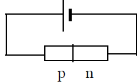A p-n junction is the region connecting the p-type semiconductor to the n-type semiconductor
• See diagram
• Electrons and holes cross the junction cancelling each other out and recombine and as a result there are no free charge carriers.

A depletion layer is therefore formed between the n-type and p-type regions and as a result a junction voltage is created.

• Electrons from n-type and holes from p-type cross the junction and recombine cancelling each other out and as a result there are no free charge carriers across this narrow insulating region.

A depletion layer is therefore formed which now acts as a ‘barrier’ between the n-type and p-type regions and as a result a junction voltage is created.

•
• The depletion layer breaks down and the diode conducts.
•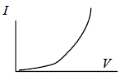The width of depletion layer gets increased and the region acts as an insulator.
• The depletion layer is overcome and as a result a large current flows.
• See diagram
• Very little current flows between 0 V and 0.6 V

If the potential difference is greater than 0.6 V a large current flows.

• A diode.
• A diode is a device that allows current to flow in one direction only.
• A rectifier
• Radio, television, computer, battery charger, mobile phone charger.
•
• Bulb B lights while bulb A does not light
• The diode near B is forward biased and therefore conducts electricity while the diode near A is reverse biased and therefore does not conduct electricity.
• LDR / light dependant resistor
•
•  Thermistor, thermometer in waterbath/oil, thermistor connected to labelled ohmmeter/(digital) multimeter
• The thermistor is connected to the ohmmeter and the value of the resistance was read from the ohmmeter.
• By allowing the apparatus to heat up over a bunsen burner.
• Label axes correctly on graph paper

Plot six points correctly
Smooth curve
Good distribution

• Range: 28↔ 32 Ω (0C–1) or Ω (K–1)
• Oil is a better conductor of heat / water contains air / (impure) water conducts electricity/good thermal contact
•
•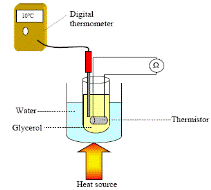See diagram
• Using an ohmmeter as shown
•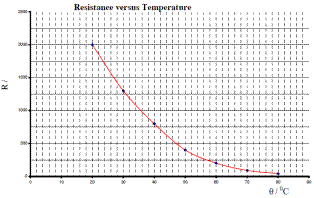• See graph
• 35 0C to 36.50 C
• Resistance goes down with increased temperature and the relationship is not linear.

•
•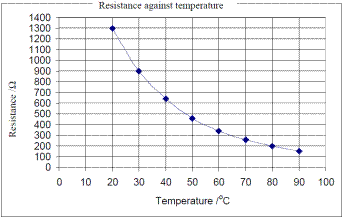Ohmmeter
• The apparatus was placed over a hot plate which heated the water and then the glycerol and the thermistor.
•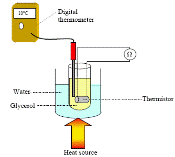Using a thermometer.
• See graph
• 35 0C.

•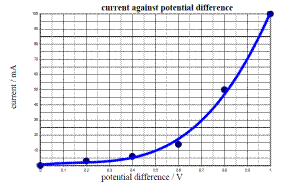• Ammeter.  It measures amps.
• Rheostat / variable resistor / potential divider

It changes the resistance which in turn changes the voltage.

• It protects the diode by limiting the current.
• See graph
• They are not proportional because the current rises rapidly after the potential difference reaches 0.6 V.

•
• See diagram
•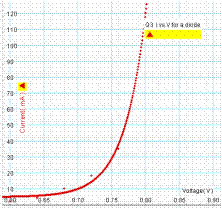Adjust rheostat / potential divider /variable power supply unit.

To measure p.d. a voltmeter was used as shown in the diagram.

• See graph
• Junction voltage = 0.60 ↔0.78 V (very difficult to be more specific).
• Reverse connections to the power supply, replace mA with μA.
• Correct shape (i.e. showing little or no current as V is increased negatively and maybe indicating a breakdown.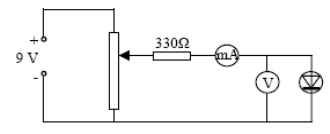Rectification
This is how a.c. is converted to d.c.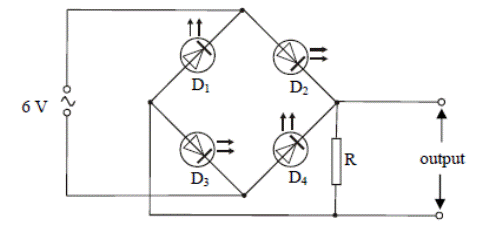• The circuit shows four light-emitting diodes connected to a resistor R and a 6 V a.c. supply of frequency 1 Hz.

What is observed when the circuit is operating?
During one second/cycle D1 and D4 flash together followed by D2 and D3 flashing together.

• Explain what is observed by referring to the circuit.

When D1 and D4 are forward biased (and so they will conduct) D2 and D3 are reverse biased

• What is observed when the frequency of the a.c. supply is increased to 50 Hz?

The diodes flash so fast that the leds appear to light continuously

• Give two functions of the resistor R.

It protects the leds from over-load and also limits the current (acts as a load resistor).

• How was the output voltage displayed?

Using a cathode ray oscilloscope or datalogger.

• Draw graphs to show the differences between the input voltage and the output voltage.

There is an a.c input and an in phase fully rectified output.

• It is noticed that the output voltage is lower than the input voltage. Explain why.

There is a voltage drop across a led ( ≈1.6 V).

Source : http://www.thephysicsteacher.ie/LC%20Physics/Student%20Notes/25.%20Semiconductors.doc

Author : not indicated on the source document of the above text

If you are the author of the text above and you not agree to share your knowledge for teaching, research, scholarship (for fair use as indicated in the United States copyrigh low) please send us an e-mail and we will remove your text quickly.

### Semiconductors

#### Semiconductors

Main page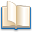Alimentation - nutritionAnimalsArchitectureArtAstrology, esoteric, parapsychology and fantasyAstronomyBiology and geneticsBotany and agricultureChemistryCourses, training, guides, handbooks and tipsCulture General and actualityDermatology, health and wellnessDictionaries and glossariesEconomics and financeEducation and pediatricsEngineering and technologyEnglish grammar and anthologyFashion and show businessFitnessFood, recipes and drinkGames, play spaceGeography, geology and environmentHistoryInformatics and computer worldJobs worldLanguagesLeisure and DIY do it yourselfLessons for studentsLiterature, biographies and journalismMathematics, geometry and statisticalMedicineMusicNotes and concept mapsPhilosophyPhotography and images - picturesPhysicsPhysiologyPsychology and psychoanalysisQuiz questions and answersReligionsRights law and political scienceScienceSociology and cultural anthropologySportSummaries and reviewsTelevision, film - movies and comicsTheater and communicationsTheses, themes and dissertationsTravel and tourist guidesUseful documents and tablesUtility, calculators and convertersWeather and meteorology

#### Semiconductors

This is the right place where find the answers to your questions like :

Who ? What ? When ? Where ? Why ? Which ? How ? What does Semiconductors mean ? Which is the meaning of Semiconductors?

##### Semiconductors physics notes

Alanpedia.com from 1998 year by year new sites and innovations